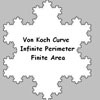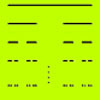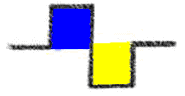#### You may also like### Sierpinski Triangle

What is the total area of the triangles remaining in the nth stage of constructing a Sierpinski Triangle? Work out the dimension of this fractal.### Von Koch Curve

Make a poster using equilateral triangles with sides 27, 9, 3 and 1 units assembled as stage 3 of the Von Koch fractal. Investigate areas & lengths when you repeat a process infinitely often.### How Many Elements Are There in the Cantor Set?

This article gives a proof of the uncountability of the Cantor set.

# Squareflake

##### Age 16 to 18Challenge Level

Jeremy of Drexel University and Andrei of Tudor Vianu National College, Bucharest, Romania sent in excellent solutions. The first half of this solution is Andrei's, the second Jeremy's.

To solve this problem, I made a table with the length and the area of the fractal at the first stages:

 Stage Length Area 0 4 1 1 $4\times2=8$ 1 2 $4\times2^2=16$ 1

 I have made the following observations: The length of the fractal at each stage is twice its length at the preceding stage. The area of the fractal is constant because the two colored areas are equal (the blue one is added, the yellow one subtracted) For the $n^{th}$ stage the length of the curve is $4 \times 2^n =2^{n+2}$ and the area is $1$ square unitNow we have the curve length for any stage $n$, and in turn, a stage $n$ for any particular curve length. \begin{eqnarray} 1,000,000 &=& 2^{n + 2}\\ n &=& log_2 (1,000,000) - 2 \\ n &\approx& 17.9 \end{eqnarray} Because 17 < n < 18 , the length must have been less than $1,000,000$ at stage $17$ and greater than $1,000,000$ at stage $18$. Thus answers the question: stage $18$. Further, we can now find the exact lengths of the curve at stage $17$ and stage $18$ to convince ourselves: $$L(17) = 2^{17 + 2} = 2^{19} = 524,288$$ which is less than $1,000,000$ $$L(18) = 2^{18 + 2} = 2^{20} = 1,048,576$$ which is greater than $1,000,000$.

The length of the fractal is $$\lim_{n\to \infty} 2^{n+2}$$ and hence the fractal curve has infinite length. This is an example of a curve of infinite length surrounding a finite area.

What is the dimension of the fractal? Solution: $d = 1.5$

Explanation: the dimension $d$ is given by the formula $n = m^d$.

$n = 8$ because each segment is broken up into 8 self-similar segments.

$m = 4$ because each of the $8$ new segments is $1/4$ the length of the original segment, and thus must be multiplied by $4$ to be the length of the original segment.

So: $8 = 4^d$ and hence $d = log_4 8 = 1.5$.